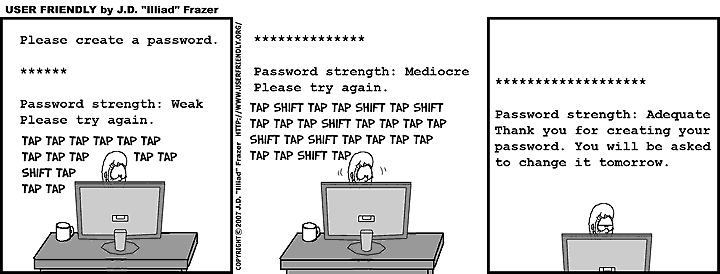# 3254. 忘记密码### 输入格式

$3$$N+2$ 行，每行一个字符串，分别表示 $s_1, s_2, \ldots, s_N$

• 对于 30% 的数据，满足：$len(T) \leq 100, \sum_i len(s_i) \leq 500$
• 对于 60% 的数据，满足：$len(T) \leq 20~000, \sum_i len(s_i) \leq 20~000$
• 对于 100% 的数据，满足：$1 \leq len(T) \leq 250~000, \sum_i len(s_i) \leq 250~000$

### 样例

Input
abcxzwwwyzxxyzzz
3
yzabcabc
ppwwwwwwwpp
wxxypp

Output
3

Input
xxxxxxxxxx
3
a
b
c

Output
-1

Input
tttttt
1
ttttttt

Output
6


### 提示

6 人解决，24 人已尝试。

14 份提交通过，共有 139 份提交。

8.5 EMB 奖励。